## Indicators

•Cited by SciELO
•Access statistics

•Similars in SciELO

## Print version ISSN 1405-5546

### Comp. y Sist. vol.21 n.4 México Oct./Dec. 2017

#### http://dx.doi.org/10.13053/cys-21-4-2787

Articles

How the Accuracy and Computational Cost of Spiking Neuron Simulation are Affected by the Time Span and Firing Rate

1 Instituto Politécnico Nacional, Centro de Investigación en Computación, Ciudad de México, Mexico svaladezg@gmail.com, hsossa@cic.ipn.mx

2 Instituto Tecnológico de León, División de Estudios de Posgrado e Investigación, Guanajuato, Mexico rsantiago66@gmail.com

Abstract:

It is known that, depending on the numerical method, the simulation accuracy of a spiking neuron increases monotonically and that the computational cost increases in a power-law complexity as the time step reduces. Moreover, the mechanism responsible for generating the action potentials also affects the accuracy and computational cost. However, little attention has been paid to how the time span and firing rate influence the simulation. This study describes how the time span and firing rate variables affect the accuracy, computational cost, and efficiency. It was found that the simulation is importantly affected by these two variables.

Keywords: Simulation; time step; time span; firing rate; numerical method; accuracy; computational cost; spiking neuron; Hodgkin-Huxley; Izhikevich; leaky integrate-and-fire

1 Introduction

Much research in recent years has focused on spiking neurons (SNs). They are mathematical models describing the action potential (AP) in a membrane patch of biological neurons [40, 54, 21, 39, 22] and are essential for several practical purposes. For instance, to simulate the generation of the electrical signals in phenomenological and detailed brain models [17, 56], to serve as elemental computational units in the third generation of artificial neural networks [53, 62], to fit experimental data from real neurons [42, 67, 19], and to build neurobiologically inspired hardware [36, 83].

An SN is defined as a non-linear system of coupled ordinary differential equations that depend on time. Due to most are hard to treat analytically, they must be solved using a numerical method through a sequence of discrete time instants [40, 2, 31, 57, 38, 8, 39, 22]. Numerical methods have an order which has to do with the number of derivative evaluations to estimate the slope to extrapolate the solution over the time instants [26, 10, 12, 24, 20, 25]. Consequently, higher order methods and small time instants produce accurate, but computationally expensive simulations, and viceversa. Furthermore, the accuracy and computational cost are the two key factors to determine whether an SN simulation is efficient or not [60, 8, 72, 61].

Theoretical studies on numerical methods deal with error estimation, existence, consistency, uniqueness, stability, and convergence [26, 57, 10, 20, 25]. Nevertheless, these qualitative studies do not explicitly determine the precise numerical method and step size to simulate a particular SN efficiently. For efficient simulation, we mean the best balance between accuracy and computational cost [2, 31, 57, 8]. Quantitative studies try to decide the precise numerical method and step size producing an effective simulation [31, 27, 68, 47, 71, 6, 59, 58, 29, 72, 81]. Finding such simulation involves comparing a testing simulation against a reference simulation. Reference simulation is implemented by the analytic or a numerical solution. The latter is performed by a high-order numerical method and a small step and can be used in any SN. The former is limited to analytically solvable models.

Not only the simulation efficiency depends on the numerical method and step size, but also on the intrinsic properties of SNs. For example, the Leaky Integrate-and-Fire model (LIF) [46, 73, 44] introduces a discontinuity error every time a spike is produced. This local error is proportional to the step size, and the global discontinuity error depends on the number of spikes generated in a simulation window . Figure 1 (top) shows a lag in the LIF spike train that depends on the simulation windows of (a) 20 ms, (b) 100 ms, and (c) 1, 000 ms.Fig. 1 Lag produced by the truncation, round-off and discontinuity errors as the time span increases. (Top) Leaky Integrate-and-Fire (LIF) model. (Middle) Izhikevich (IZH) model. (Bottom) Hodgkin-Huxley (HH) model. The black line corresponds to a benchmark simulation and the dark blue to a testing simulation. The light blue line in g-i is the HH with the rate constants stored in tables. The Fourth-Order Runge-Kutta (RK4) method with a step size of 0.0001 ms was employed for the reference simulations and the Forward Euler (FE) with a step size of 0.05 ms for the testing ones. Each spiking neuron gave a firing rate of 70 Hz

The simulation in blue is a testing simulation that is compared against a reference simulation in black. The lag is because of the combination of the errors mentioned. In contrast, the Izhikevich (IZH)  introduces two discontinuity errors: one for the voltage variable and the other for the recovery variable, see Eq. (5). The error in the recovery variable depends on the error of the voltage variable . As opposed to other bi-dimensional models (e.g., the quartic  or the adaptive exponential ), the IZH adaptation variable blows up without a threshold value .

This makes the model sensitive to threshold values and, as a consequence, the step size must be small to avoid an alteration in the system dynamics [77, 79].This agrees with practical works where a high-order numerical method and a small step were necessary to obtain an accurate implementation [35, 72, 81]. Figure 1 (middle) depicts the considerable lag generated by the IZH as the simulation window increases. In Fig. 1f, it is seen that the lag is such that the penultimate spike (blue line) tends to match the last spike (black line). On the other hand, continuous models, such as the Hodgkin-Huxley (HH) , do not introduce any discontinuity error because the spike generation is based on continuous functions. Figure 1 (bottom) shows that the lag is negligible at any of the simulation windows. If the rate function values are pre-stored in tables (as suggested by Hodgkin and Huxley in ), the lag is slightly increased, see the light blue line in Fig. 1i. This lag is for the reason that the continuous rate functions are discretized when stored in tables. Testing and reference simulations in Fig. 1 were solved by the same numerical methods and step sizes. The reference simulation for each SN was implemented by a high-order numerical method and a small step. The firing rate was similar among them.

Although earlier works have focused on how the simulation is affected by the numerical method, step size and spike generation mechanisms, little attention has been paid to the time span and firing rate influence. Depending on the numerical method and SN, the accuracy increases monotonically as the step size reduces [61, 81, 34, 72, 29]. This is consistent with the theory of numerical methods where the solution provided by a convergent method approximates the exact solution as the step size tends to zero [26, 10, 24, 20, 25]. What is more, quantitatively, the accuracy behavior is not yet well understood given the wide range of measures to calculate the precision, the large number of SNs and numerical methods in the literature; and the infinite number of step sizes to choose from.

On the contrary, it is known that the computational cost increases in a power-law complexity as the step sizes reduces . This behavior is also dependent on the numerical method and SN. The most studied aspect of SN simulation is the discontinuity error produced by the resetting mechanism to generate the AP that plenty of algorithms have emerged, mainly that of the LIF [27, 71, 47, 52, 6, 59, 66, 58, 29] and IZH [80, 79, 34]. Even though there are several open issues in the single SN simulation, this paper focuses on the time span and firing rate influence. It is thought that the firing rate changes the accuracy that some studies [50, 72, 81] have included it, but its impact has not been measured. The influence of the time span variability has been ignored and, in consequence, remains unknown. Most current works have carried out simulations just on a single time span [31, 27, 68, 47, 71, 52, 6, 59, 66, 58, 79, 29, 72, 34, 81].

This study describes how the accuracy, computational cost, and efficiency of an SN simulation are influenced by the time span and firing rate variability. We followed and extended the studies carried out in  and  where three important SNs were investigated: the LIF, IZH, and HH. In those works, each SN was solved with three different numerical methods and five different time steps.

Also, the SNs were stimulated with a constant input current to produce one of the simplest neuro-computational properties: the regular firing pattern . Those studies analyzed three different firing rates. A numerical solution was implemented as a benchmark simulation since the exact solution is unknown for the IZH and HH. However, they used a single time span, and the influence of the firing rate to the simulation was not studied. This work examines the influence of other simulation windows not considered in the previous researches and studies the impact of the firing rates on the simulation. An important difference between  and  are the measures they used to test the accuracy, computational cost, and efficiency. These measures will be discussed in Section 4.

On the whole, it was found that the simulation efficiency and accuracy fall linearly as the simulation window increases. The efficiency and accuracy stay constant for some firing rates and varies linearly or no-linearly for others depending on the SN model. The computational cost increases linearly with the time span and remains constant with the firing rate. All of these suggest that not only the numerical method, step size and spike generation mechanisms affect the SN simulation, but also the time span and firing rate. In previous studies, these two parameters have been considered irrelevant to the SN simulation.

In his influential article , Izhikevich stated that 1) the HH is prohibitive, 2) the IZH is as efficient as the LIF, and 3) the IZH is more efficient than the HH. Until now, these statements have had a profound impact in the SN research area. Conversely, other authors have suggested these statements could be wrong [35, 51, 77, 72, 49, 81]. This paper gives new evidence in line with them, but for the firing rate and time span variation. When the efficient SN simulations were compared, the HH showed the highest efficiency level for any time span and firing rate, by contrast, the IZH showed the lowest. A similar behavior was observed for the accuracy in most cases. In all cases, the IZH computational cost is close to that of the HH and HH-T rather than that of the LIF.

This article is organized as follows. Section 2 describes the SNs models. The numerical methods are given in Section 3. Section 4 shows the measures to test the level of the SN simulations. Section 5 focuses on the results. Section 6 makes the conclusions.

2 Spiking Neurons

There is a significant number of SNs [22, 39]. For simplicity, the LIF, IZH, and HH were reviewed because they are used in many simulation studies [38, 74, 67, 50, 72, 81, 82, 61] and to re-test the Izhikevich affirmations above mentioned. Also, these SNs are elemental models from which reductions or extension are proposed. The LIF is the simplest representation of the spike generation that is widely used owing to its low computational cost and implementation ease [1, 9].

The HH is the most biologically plausible model that works at the particle dynamics and electrical current levels. The HH also can be seen as a generalization of the LIF where the LIF works with passive and the HH with active conductances . The IZH is a dimensional reduction of the HH  that can reproduce twenty neuro-computational properties . On the contrary, the LIF reproduces three properties and the HH at least seventeen . The IZH can be an integrator as the LIF or a resonator as the HH .

2.1 Leaky Integrate-and-Fire

Taking the notation in , the LIF [46, 73, 44] is defined as:

where V, Vth, and Vrest are the trans-membrane, threshold and resting potentials, respectively. R is the trans-membrane resistance; I is the stimulation current, and τ = RC is the membrane time constant (here C is the trans-membrane capacitance).

2.2 Hodgkin-Huxley

Hodgkin and Huxley  defined their Nobel-Prize winning model as:

where V is the trans-membrane voltage, C = 1 µF/cm2 is the membrane capacitance, and I is the stimulation current. EK = −12 mV and ENa = 115 mV are equilibrium potentials for the K and Na ions, respectively.

EL = 10.6 mV is the reversal potential for chloride and other ions.,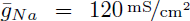and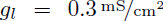are the conductance for the K, Na, and other ions, respectively. Equilibrium potentials, conductances, and capacitances are constant values defined in . α’s and β’s are rate constants defined as:

α’s and β’s definition was taken from .

2.3 Izhikevich

The IZH  is modeled as:

Here V is the trans-membrane potential, n is a recovery variable, I is the stimulation current, a is a time scale for n, b is the sensitivity to the sub-threshold fluctuations of n, c is a reset value of V , and d is a reset value for n.

2.4 Configuration

The constant stimulation currents and simulation parameters were taken from  and . The passive parameters for the LIF were C = 5.0675 nF, R = 8.22 MΩ, Vth = 30 mV and Vrest = 0 mV. Each spike was followed by a refractory period of tref = 5 ms. The input currents to generate the firing rates of 70, 90, and 120 Hz were 18, 28, and 55 µA/cm2 , respectively.

The values for the IZH were a = 0.02, b = 0.2, c = −65 and d = 2 to generate a regular spiking . The IZH was stimulated with the adimensional constant currents of 13, 15, and 19 to produce the firing rates of 70, 90, and 120 Hz.

The HH was stimulated with the constant currents of 13, 20, and 50 µA/cm2 to produce the firing rates of 70, 90, and 120 Hz. Applying the I’Hôpital rule, we defined αn = 0.1 for V = 10 and αm = 1.0 for V = 25 αn αn and αm in Eq. (4) are indeterminate for those values . According to , the initial values for n, m, and h are:

where α’s and β’s are evaluated for V0.

The HH was simulated by the α’s and β’s pre-stored in tables at intervals of 1 mV (as suggested by Hodgkin and Huxley  and implemented in [30, 72, 81]). In the rest of this work, the HH using tables will be referenced as HH-T and the other as HH.

3 Numerical Methods

Many numerical methods, classified as time- and event-driven techniques, can be chosen to solve an SN [8, 61]. Time-driven techniques include the exponential integrators and Runge-Kutta methods, which can be implicit or explicit [26, 10, 24, 20, 25]. Implicit numerical methods are more complicated for implementation than the explicit given the use of a current and a next state to calculate the solution approximation. Therefore, ODEs must be algebraically rearranged to join the next state variables. Event-driven techniques are exclusive to analytically solvable SNs for the reason that the exact solution is necessary to calculate the event (spike) timing [8, 61].

For the sake of simplicity, three explicit numerical methods were selected: the Forward Euler (FE) , the Fourth-Order Runge-Kutta (RK4)  and the Exponential Euler (EE) [65, 55]. Other authors have found that the LIF, IZH, and HH are solved by these methods [13, 16, 5, 28, 37, 38, 35, 63, 69, 4, 72, 81].

Apart from that, the FE is the simplest numerical method, and the RK4 is one of the most accurate [26, 10, 24, 20, 25]. The EE is the default integrator in many neural simulation software , and this is thought to produce efficient solutions in neural systems [5, 31, 23]. The EE is the simplest exponential integrator . Making a differential equation an arbitrary function, i. e. dy/dt = f(t, y), the solution for y in the next time update yn+1 can be extrapolated from a previous value yn by some integration technique. Based on that, the FE, RK4, and EE can be derived.

3.1 Forward Euler

As defined by Euler , the FE is:

where

where h is the step size, which is a fixed value during the entire simulation.

3.2 Fourth-Order Runge-Kutta

Kutta  expressed his method as:

where

are slope evaluations for f(t, y).

3.3 Exponential Euler

Exponential integrators date back to . MacGregor  was one of the first in applying exponential integrators to neural modeling. As the ODEs of the SNs studied here are of first-order, they can be represented as:

where A and B are arbitrary functions of t. If A and B are constant during the interval h, then the solution of Eq. (11) is given by:

It is noteworthy to mention that, as in , when the SNs and the numerical method were put together the resulting expressions were rearranged to reduce the computational cost.

3.4 Benchmark Scheme

As there is not an analytic solution for the IZH and HH, the benchmark scheme defined in  and  was employed. In this scheme, several testing simulations were compared to a reference simulation. The selected numerical algorithm and step size were those that resulted in the maximum accuracy and minimum computational cost possible.

In this work, each SN (i.e., the LIF, IZH, HH-T, and HH) was solved by each numerical method (i.e., the FE, RK4, and EE) with the step sizes of 0.0001, 0.001, 0.01, 0.1, and 1 ms. The reference simulation for each SN was implemented using the RK4 with a step size of 0.0001 ms [72, 81]. Each SN was stimulated by three different constant currents given in Subsection 2.4 to produce a regular firing rate of approximately 70, 90, and 120 Hz [72, 81]. The regular firing pattern is one of the simplest neuro-computational properties an SN produces. Apart from the time span of 1000 ms studied in  and , each simulation was run over the windows of 10 and 100 ms.

4 Accuracy and Computational Cost Measures

There are several aspects to determine whether an SN simulation is efficient or not. As in , the simplest aspects were analyzed: the voltage time course and the spike-timing. The computational cost can be measured by the Floating Point Operations (FLOPS) or the CPU execution time. The latter was used.

The Root Mean Square Error (RMSE) is one of the simplest and commonest measures to calculate the difference between the voltage time course of a testing and a reference simulation. Nevertheless, the RMSE is not a reliable measure of accuracy [84, 11]. The RMSE increases the influence of large differences in the total error. For this reason, the Voltage Coincidence Factor (VCF)  was used. Contrary to the RMSE, the VCF reduces the influence of large differences giving more importance to the similarities. Whereas in  the RMSE was measured on a single spike, in  the VCF was computed on the entire spike train. This is important because, as already mentioned, the various errors produce a lag increment with the time span (see Fig. 1). So, the voltage time course for the whole simulation window was measured.

The Spike Coincidence Factor (SCF) [41, 42, 43] was used to measure the spike-timing precision of a testing simulation. In , the spike-timing precision was measured by the second and last spike-timing that were later used to calculate a frequency error, thereby eliminating the precise spike-timing. Spike-timing is thought as a critical factor for complex neural computations in real neurons and artificial learning algorithms, including functional and morphological plasticity in learning and memory, coincidence detection, directional selectivity, synaptic integration, synchronization; and AP initiation and conduction [48, 85, 15, 75]. Some artificial algorithms where the precise spike-timing is a key factor include the SpikeProp  and ReSume [64, 76].

The common measure of computational cost is the CPU time. Other studies have used the Floating Point Operations (FLOPS). Nonetheless, the CPU time is more precise than FLOPS because FLOPS are only a qualitative description of the computational cost. While the FLOPS were utilized in , the Computational Cost Factor (CCF) was used in . The CCF is a normalized measure that is based on the CPU execution time.

The Global Performance Factor (GPF) was used to find the overall efficacy of an SN implementation . The GPF is a weighted sum that scalarizes the SCF, VCF and the CCF into a single objective function. The weighted sum is the commonest and simplest approach when there exists a trade-off between several objectives .

4.1 Voltage Coincidence Factor

The VCF determines whether the voltage time course in a testing simulation is optimal against that of a reference simulation . The VCF is set as:

where T is the simulation time span, x is the voltage difference between the testing and benchmark simulations divided by the precision tolerance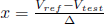and g is a generic functionthat goes from 0 to 1. Accuracy tolerance was defined as ∆ = 15 mV [72, 81]. The VCF is a normalized measure that gives 1 for coincident simulations and 0 for non-coincident.

4.2 Spike Coincidence Factor

The SCF allows determining the spike-timing level of a testing simulation against a reference [43, 41, 42]. This factor is defined as the number of spike coincidences minus the number of related correspondences divided by the total spike number in both simulations. More precisely:

where Nref and Ntest are the spike number in the reference and testing simulations, respectively. Ncoinc is the number of coinciding spikes in both simulations with a precision of ∆ = 2 ms, the AP duration in cortical neurons [41, 42].is the expected number of coincidences generated by a homogeneous Poisson process with an occurrence rate of(where T is the length of the simulation) at the same rate of occurrence as the testing simulation. If and only if Ncoinc = Nref = Ntest, the factor α = (1 − 2v∆)−1 normalizes the coincidence to a maximum value of 1. A Poisson process with the same rate of occurrence as the testing simulation produces on average 0.

This implies that the benchmark simulation is better than the testing simulation because there is no correlation between the spike-timing in both simulations as the testing spike train was random. A negative value can be obtained, in which case, there is a negative correlation between the two spike trains. The denominator 1/2 (Nref + Ntest) ensures that the average firing rate of the two spike trains is the same. Although the SCF depends on the tolerance ∆, there is no critical dependence to ∆ = 2 ms because its value is constant for 1 ≤ ∆ ≤ 12 ms . More importantly, the SCF is accurate for constant input currents .

4.3 Computational Cost Factor

The CCF determines the level of computational cost for a testing simulation . This factor is based on the CPU time of both testing and reference simulations, i.e.:

where xref is the CPU execution time of the benchmark simulation and xtest is the CPU time from the testing simulation.

It is supposed that the reference simulation consumes more or at least the same CPU time than any testing simulation (i.e., xref xtest ) since the reference simulation is configured with the highest order method and the smallest step size possible. In the upper boundary, if the computational cost in the testing simulation is equal to that of the reference simulation (i.e., xref = xtest ), the ratiogives 1.

In the hypothetical and lower bound case where the testing simulation does not spend any fraction of time (i.e., xtest = 0), the ratiogives 0. Thus, the ratiois a function that is minimized as the testing CPU time decreases.

It is better either to maximize or to minimize all factors to work easier with mixed objectives (i.e., accuracy maximization and computational cost minimization). By the duality principle in optimization problems , the ratiowas converted from a minimization function to a maximization one by subtracting it from 1.

In this way, if xref = xtest then CCF = 0, which means that the testing simulation is not optimal in computational cost. If xtest → 0 then CCF → 1, which indicates that the testing simulation tends to be optimal. CCF = 1 is impossible to reach as none simulation is performed without expending any fraction of CPU time. Owing to the simulation time fluctuates for each run, the CPU time was recorded as the average of 20 runs.

4.4 Global Performance Factor

This factor is a weighted sum:

where k is the number of factors Fi to include in the overall performance, and i is an index. There are three factors: F1 = CCF , F2 = SCF , and F3 = VCF . Hence, Equation (16) becomes

The efficient SN simulation will be that with the maximum GPF value. The weights for each factor were assigned according to  and following the recommendations in . They suggest that the maximum weights would be given to the most important factors. The maximum weight has been paid to the CCF due to it is imperative to get the numerical method and time step size producing the minimum computational cost possible. For simulation accuracy, it was decided on the SCF with a higher weight than VCF because of the spike-timing importance in neural computation and to the necessity to guarantee the firing rate coincidence.

5 Results

Previous works have paid little attention to the consequences of the time span and firing rate for the SN simulation. This work describes how the simulation is affected by these two aspects. To achieve such an objective, we limited our research to three relevant SNs: the LIF, IZH, and HH. Each one was stimulated with three different constant currents to produce a regular firing rate of approximately 70, 90, and 120 Hz. These SNs were solved by three commonly used numerical methods in neural simulation: the FE, RK4, and EE. Each numerical method used the time steps of 0.0001, 0.001, 0.01, 0.1, and 1 ms. Simulations were run over three different time spans: 10, 100, and 1, 000 ms.

The accuracy was measured by considering the simplest aspects of an SN simulation: the voltage time course and the spike-timing. The accuracy level between a testing and a reference simulation was calculated by the VCF and the SCF. The VCF and SCF give a normalized value of the coincidence level in two simulations for the voltage time course and spike-timing, respectively. On the other hand, the computational cost was measured by the CCF, which gives a normalized CPU execution time for the testing simulation when compared to the reference simulation. The GPF was used to determine the efficiency level of an SN simulation. The GPF is a weighted sum that scalarizes the VCF, SCF, and CCF into a single decision function. These factors are more accurate than those used in  and other studies.

As there is no analytic solution for the IZH and HH, the benchmark scheme in  and  was used. Several testing simulations using different numerical methods and step sizes were compared to the reference simulation. The reference simulation for each SN was implemented by the RK4 with a step size of 0.0001 ms. The numerical algorithm and step size giving an efficient simulation were those that resulted in the maximum GPF. The GPF, VCF, SCF, and CCF were studied for the different time spans and firing rates mentioned.

5.1 Accuracy

Table 1 shows the SCF for a firing rate of 70 Hz over a time span of 10 ms. It is seen that the LIF produces satisfactory temporal coincidences at any numerical method and step size. Remember that SCF = 1 means a timing coincidence. The spike-timing of the IZH, HH-T, and HH is imprecise when a step size of 1 ms is used. As a result, the LIF produces a higher spike-timing precision than the other SNs.

Table 1 Spike Coincidence Factor (SCF) for a firing rate of 70 Hz over a 10 ms time span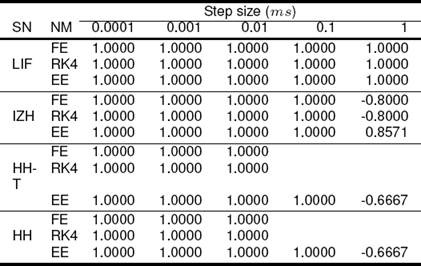Table 2 reports the SCF for the same firing rate reported in Table 1 but over a time span of 100 ms. Comparing Tables 1 and 2 reveals that the SCF is reduced with the time span increment when a time step of 0.1 and 1 ms is used. Figure 1 confirms this behavior where the lag in the spike-timing increases as the temporal window increases. The following paragraph discusses the VCF results, which give a more precise value to appreciate the accuracy behavior.

Table 2 Spike Coincidence Factor (SCF) for a firing rate of 70 Hz over a 100 ms time spanTables 3 and 4 lists the VCF for a firing rate of 70 Hz over a time span of 10 and 100 ms, respectively. It is demonstrated that the VCF is also reduced as the time span is increased from 10 to 100 ms. This is visible in Fig. 1 where the voltage time course suffers from a mismatch as the time window varies.

Table 3 Voltage Coincidence Factor (VCF) for a firing rate of 70 Hz over a 10 ms time spanTable 4 Voltage Coincidence Factor (VCF) for a firing rate of 70 Hz over a 100 ms time spanFigure 2 plots the VCF given in Table 3 for the (a) FE, (b) RK4, and (c) EE. As illustrated, the voltage accuracy increases monotonically as the step size reduces.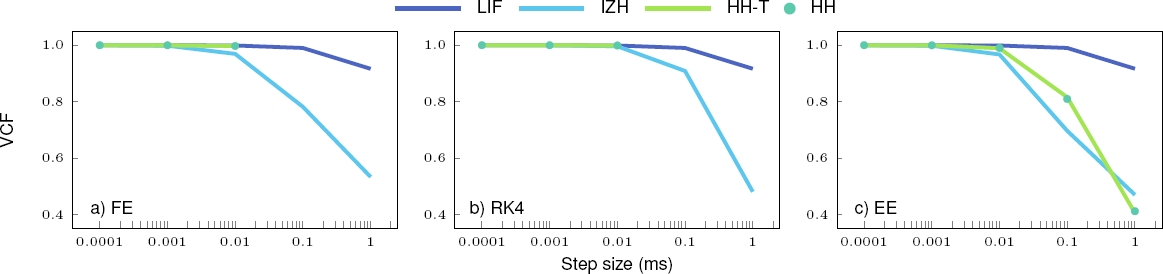Fig. 2 The Voltage Coincidence Factor (VCF) given in Table 3 in function of the step size for a firing rate of 70 Hz and a time span of 10 ms. Numerical methods: (a) FE=Forward Euler, (b) RK4=Fourth-Order Runge-Kutta and (c) EE=Exponential Euler. Spiking neurons: LIF=Leaky Integrate-and-Fire, IZH=Izhikevich, HH=Hodgkin-Huxley and HH-T=HH using tables

The same happens to the other time spans and firing rates (data not shown). This agrees with the theory where the solution provided by a convergent numerical method approximates the exact solution as the step size tends to zero. Figure 2 also indicates that the IZH is the least accurate and the LIF the most one for a firing rate of 70 Hz and a time span of 10 ms. The same happens for 70 Hz and a time span of 100 ms (see Table 4).

Table 5 lists the spike number in a time span of 10 ms for a firing rate of 70 Hz. It is seen that any numerical method and step size produce the same spikes compared to the reference simulation (RK4 with a step size of 0.0001 ms), except with the IZH. There is a different spike number produced by the step size of 1 ms. This confirms the IZH inaccuracy.

Table 5 Spike number for a firing rate of 70 Hz over a 10 ms time span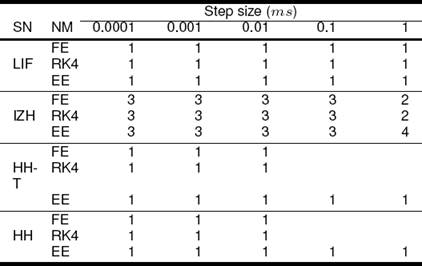Table 6 displays the spike number, but in a time span of 100 ms. Inspection of Tables 5 and 6 shows that, as the time span increases, the spike number difference also increases. This is in line with the VCF reduction in Tables 1-4.

Table 6 Spike number for a firing rate of 70 Hz over a 100 ms time spanIt is thought that the spike number or firing rate is enough to determine the accuracy level. The firing rate does not ensure that the voltage and the spike-timing are coincident. In any case, the coincidence in the spike number is a necessary but not a sufficient condition.

This is taken into account in Eq. (14) where the expression 1/2 (Nref + Ntest) ensures that at least there is the same spike number in the testing and reference simulations. We now know an accurate simulation will be produced by those numerical methods and step sizes giving the same spike number to that of the reference simulation.

This is seen in Tables 5 and 6 where the smallest step sizes give the same spike number for any SN. As theoretically expected, the most accurate simulations are produced by the smallest step sizes and highest order methods, as detailed in Tables 1-6.

Our evidence suggests that the time span also affects the simulation accuracy (i.e., the SCF, VCF, and spike number). It may be reasonable to suppose a correlation between the SCF, VCF and spike number. The spike number must be the same in the testing and reference simulations to be accurate in both the voltage time course and spike-timing. The spike-timing in the two simulations must match to be coincident with the voltage time course, and vice-versa. Up to this point, an efficient simulation cannot be found given that the computational cost influence has not been considered.

5.2 Computational Cost

Tables 7 and 8 contain the average CPU time for 20 runs over a time span of 10 and 100 ms, respectively. The firing rate for both temporal windows was 70 Hz. As expected, the computational cost is markedly affected by the temporal window where the highest values are produced by the larger simulation window.

Table 7 CPU execution time (s) to produce a firing rate of 70 Hz over a 10 ms time spanTable 8 CPU execution time (s) to produce a firing rate of 70 Hz over a 100 ms time spanAlso, the numerical method with higher order and smaller step size provide higher CPU times. As anticipated in , the CPU time follows a power-law behavior with the step size. Figure 3 (top) shows the power-law distribution of data in Table 7. A power-law distribution can be converted to a linear equivalent form by applying the natural logarithm on data (Fig. 3 (bottom)). Inspection of Fig. 3 indicates that the most expensive is the HH, followed by the HH-T, IZH, and LIF. In addition, the RK4 is more expensive than both the FE and EE, which are similar in computational cost. The same is true for a firing rate of 70 Hz and a simulation window of 100 ms. In , a procedure to fit the CPU time data to a power-law function has been proposed. This function can be a reliable measure of computational cost.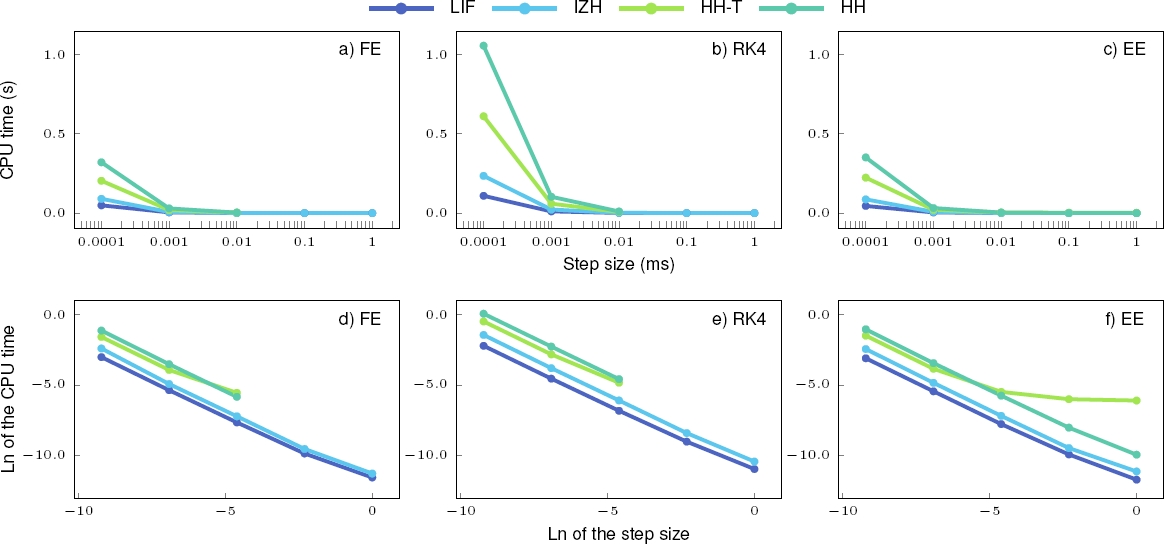Fig. 3 Power-law distribution (top) and its linearization (bottom) for various spiking neurons: (a and e) Leaky Integrate-and-Fire (LIF), (b and f) Izhikevich (IZH), (c and g) Hodgkin-Huxley (HH) and (d and h) HH using tables (HH-T). Numerical methods: FE=Forward Euler, RK4=Fourth-Order Runge-Kutta, and EE=Exponential Euler. The CPU time is an average of 20 runs

Tables 9 and 10 present the CCF, which is based on the ratio between each testing CPU time and the reference CPU time shown in Tables 7 and 8. The CCF stays similar with the simulation window. Also, the CCF is higher for simulations carried out by low-order numerical methods and large step sizes. Remember that the CCF is the dual function (maximization) of the computational cost minimization. So, the reference simulation gives CCF = 0 to the most expensive (i.e., the RK4 with a step of 0.0001 ms).

Table 9 Computational Cost Factor (CCF) for a firing rate of 70 Hz over a 10 ms time spanTable 10 Computational Cost Factor (CCF) for a firing rate of 70 Hz over a 100 ms time spanNow, we are in a position to select a numerical method and step size leading a balanced simulation through the GPF.

5.3 Global Performance Factor

The accuracy (i.e., the SCF and VCF) and computational cost (i.e., the CCF) separately do not determine which numerical method and step size produce an efficient simulation. For that purpose, the GPF considers the contribution of these three factors. Table 11 lists the GPF, which is based on data in Tables 1, 3, and 9, for a firing rate of 70 Hz and a time span of 10 ms. The maximum value (gray cells) for each SN indicates the best compromise between computational cost and accuracy according to the balance function defined in Eq. (17). These results prove that the LIF is efficiently simulated by the EE with a step size of 0.1 ms and the IZH needs the RK4 with a step size of 0.01 ms. The FE and a step size of 0.01 ms produce the highest efficiency for both the HH-T and HH. Table 12 gives the GPF, which is based on data in Tables 2, 4, and 10, for a time span of 100 ms and similar results are seen. The only difference is the reduction in the step size for the LIF from 0.1 ms to 0.01 ms.

Table 11 Global Performance Factor (GPF) for a firing rate of 70 Hz over a 10 ms time spanTable 12 Global Performance Factor (GPF) for a firing rate of 70 Hz over a 100 ms time spanUp to this point, the time spans of 10 and 100 for a firing rate of 70 Hz were analyzed. A similar procedure was carried out to obtain the efficient simulations for the time span of 1, 000 ms and the frequencies of 90, and 120 Hz. Table 13 summarizes the numerical method and step size giving an efficient simulation (maximum GPF) for each pair window-frequency. It is seen that the LIF is best simulated by the EE with a step size of 0.01 ms for the windows of 100 and 1, 000 ms, and with a step size of 0.1 ms for the simulation window of 10 ms.

Table 13 Numerical method (NM) and step size selection for optimal simulation on different firing rates and time spansThe IZH produces the best efficiency with the RK4 and a step of 0.01 ms for any time span and firing rate. It is apparent that the FE and a step length of 0.01 ms increase the HH-T and HH simulation efficiency. The RK4 could be necessary when a simulation window of 1, 000 ms is used for the HH and HH-T. Table 13 suggests that neither the time span nor the firing rate could affect the final numerical method and step size selection. However, it will be demonstrated that the efficiency (i.e., the GPF), accuracy (i.e., the SCF and VCF) and computational cost (i.e., the CCF) levels do are affected by the time span and firing rate.

The time span and the firing rate impact can be seen through the GPF. From a comparison of Tables 11 and 12, the maximum GPF varies as the simulation window changes. The variation within the simulation windows and firing rates is better seen if the maximum GPFs giving the results in Table 13 are placed in a graphical depiction (see Fig. 4).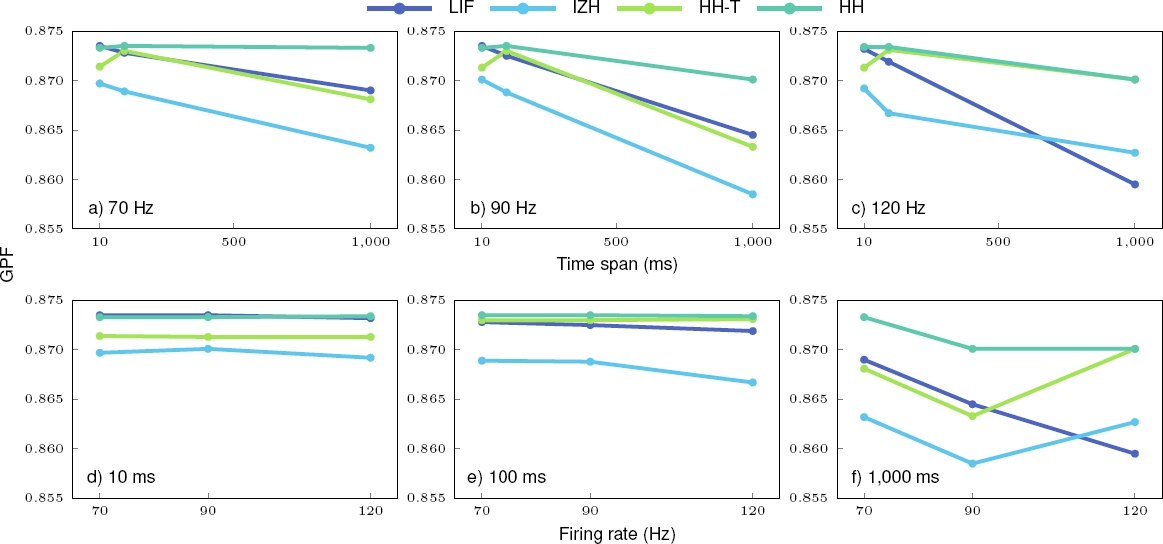Fig. 4 The maximum Global Performance Factor (GPF) as a function of the time span (top) and firing rate (bottom). Spiking neurons: LIF=Leaky Integrate-and-Fire, IZH=Izhikevich, HH=Hodgkin-Huxley, and HH-T=HH using tables

Figure 4 (top) shows that the efficiency (maximum GPF) reduces linearly as the simulation window increases. The LIF and IZH efficiency decreases linearly with the time span. But, the IZH efficiency presents a little negative inflection from 10 to 100 ms for a firing rate of 120 Hz. It should be noted that the HH-T and HH efficiency barely increases from 10 to 100 ms at all firing rates. This last is contrary to the IZH negative inflection at the same time span. The efficiency variation from 10 to 1, 000 ms is little affected (0 − 1.57 %, depending on the SN and firing rate).

According to Fig. 4 (bottom), the efficiency remains constant for the frequencies of 70 and 90 Hz, whereas for a firing rate of 120 Hz the efficiency changes depending on the SN. The LIF efficiency falls linearly with the simulation window increment and the IZH, HH-T, and HH efficiency increases from 90 to 120 Hz. The efficiency change from 70 to 120 Hz is little affected (i.e., 0.11−1.09 %, depending on the SN and simulation window). Hence, the efficiency varies more within the time spans than within the firing rates.

It is observed from Fig. 4 that the HH is the most efficient. In most cases, the IZH is the less efficient, except for a firing rate of 120 Hz and a time span of 1, 000 ms. The LIF and HH-T are closer considering the firing rates of 70 and 90 Hz and the time spans of 100 and 1, 000 ms.

5.4 A Single Efficient Simulation

To choose a single numerical method and step size producing efficient simulation for any simulation window and firing rate studied here, the GPF over all the simulation windows and firing rates was averaged. The results are given in Table 14 where the maximum averaged GPFs (gray cells) reveal that the LIF is efficiently simulated by the EE (or even by the FE since it produces virtually the same result) and the IZH by the RK4. The HH-T and HH are best simulated by the FE. All SNs are efficiently simulated with a step size of 0.01 ms. These results can also be inferred from Table 13. It is also observed from Table 14 that the HH presents the highest efficiency (0.8721), followed by the LIF (0.8697), HH-T (0.8691), and IZH (0.8664).

Table 14 Average of the Global Performance Factor (GPF) over all pairs window-frequency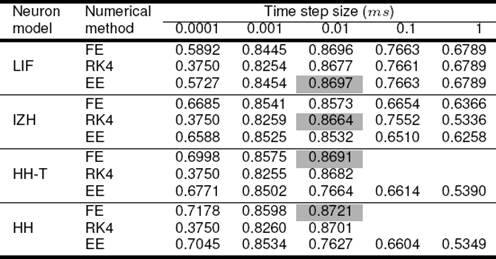Figure 5 displays the VCF as a function of the time span (top) and firing rate (bottom) for the single efficient simulation. Figure 5 (top) demonstrates that the accuracy diminishes as the simulation time span reduces where in most cases the HH is the most accurate. The accuracy reduction from 10 to 1, 000 ms is 2.37 − 9.59 %, 5.22 − 9.14 %, and 5.92 − 9.87 % for the LIF, IZH, and HH-T, respectively. The HH increases 0.17 % for 70 Hz and decreases 2.85 − 6.13 % for the other frequencies. As shown in Fig. 5 (bottom), not necessarily the accuracy reduces with the firing rate.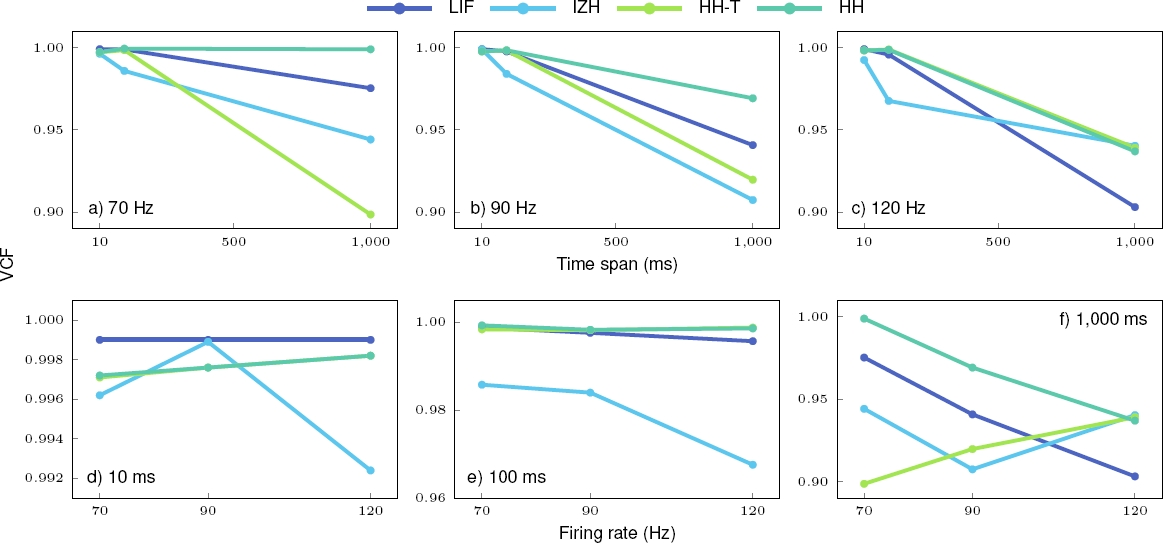Fig. 5 The Voltage Coincidence Factor (VCF) as a function of the time span (top) and firing rate (bottom). Spiking neurons: LIF=Leaky Integrate-and-Fire, IZH=Izhikevich, HH=Hodgkin-Huxley, and HH-T=HH using tables

In particular, the IZH accuracy increases from 70 to 90 Hz over 10 ms and 90 to 120 Hz over 1, 000 ms. Surprisingly, the HH-T accuracy increases linearly with the frequency for a temporal window of 1, 000 ms. The accuracy decrement from 70 to 120 Hz is 0 − 7.40 % and 1.84 − 0.40 % for the LIF and IZH, respectively. The HH-T increases 0.04 − 4.30 %. The HH increases 0.09 % for 70 Hz and reduces 0.07 − 6.19 % from 90 to 120 Hz.

From Fig. 6, it can be seen the CPU time as a function of the time span (top) and firing rate (bottom) for the single efficient simulation. The computational cost increases linearly with the time span (Fig. 6 (top)) and remains nearly constant with the firing rate (Fig. 6 (bottom)). The increment from 10 to 1, 000 ms is 14, 708 − 15, 779 %, 10, 977 − 12, 078 %, 5, 307 − 6, 612 %, and 11, 131 − 11, 507 % for the LIF, IZH, HH-T, and HH, respectively. The HH is the most expensive, followed by the IZH, HH-T, and LIF. It seems probable that the HH is not prohibitive as Izhikevich stated in his influential paper .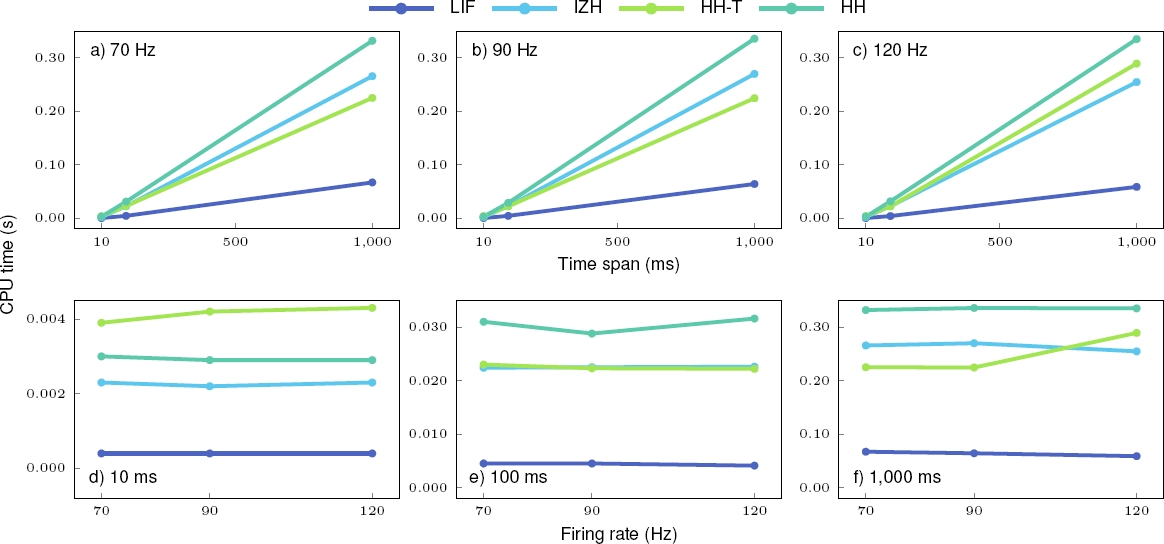Fig. 6 The CPU time as a function of the time span (top) and firing rate (bottom). Spiking neurons: LIF=Leaky Integrate-and-Fire, IZH=Izhikevich, HH=Hodgkin-Huxley, and HH-T=HH using tables

From the FLOPS mentioned by Izhikevich in  (i.e., 13 FLOPS for the IZH and 1, 200 for the HH), the HH is 9, 130 % more expensive than the IZH. From Fig. 6, the HH is 24 − 40 % more expensive than the IZH depending on the simulation window and firing rate. Figure 6 shows that the IZH CPU time is nearer to both the HH-T and HH, instead of the LIF. The IZH and HH-T are close in computational cost.

6 Conclusions

Prior work paid little attention to the simulation window and firing rate consequences for the SN simulation. In this study, we reviewed the LIF, IZH, and HH models for the simulation windows of 10, 100, and 1, 000 ms and the firing rates of 70, 90, and 120 Hz. SNs were solved by the FE, RK4, and EE with the step sizes of 0.0001, 0.001, 0.01, 0.1, and 1 ms.

The accuracy of the voltage time course and spike-timing were measured by the SCF and VCF, respectively. The computational cost was measured by the CPU time through the CCF. The GPF, which is a weighted sum considering the SCF, VCF, and CCF contribution, was used to determine the efficiency level. These factors were studied for the time spans and firing rates mentioned.

It was found that in all cases, the SN simulation is affected by the time span and firing rate. The efficiency reduces linearly as the simulation window increases and remains constant for the frequencies of 70 and 90 Hz. For a firing rate of 120 Hz, the efficiency changes depending on the SN. In any case, the efficiency variation is little affected. The HH is the most efficient of all SNs. In most cases, the IZH is the less efficient, except for a firing rate of 120 Hz and a time span of 1, 000 ms. The LIF and HH-T are closer in efficiency for the firing rates of 70 and 90 Hz and the time spans of 100 and 1, 000 ms.

The accuracy diminishes as the simulation time span reduces where in most cases the HH is the most accurate. The reduction is enough to consider an important accurate variation with the simulation window. The accuracy can decline or rise depending on the SN. The accuracy changes more with the time span than with the firing rate.

The computational cost increases drastically in a linear way with the time span and remains practically constant with the firing rate. The HH is the most expensive, followed by the IZH, HH-T, and LIF. The IZH computational cost is nearer to both the HH-T and HH rather than to the LIF. The IZH and HH-T are close in computational cost. It is suggested using the EE numerical method to efficiently solve the LIF, the RK4 for the IZH, and the FE for the HH and HH-T (all numerical algorithms with a step size of 0.01 ms). Also, we recommend using the HH-T rather than the HH.

Our results expand prior work demonstrating that the accuracy increases monotonically and the computational cost increases in a power-law complexity as the step size increases. Also, previous works have shown that the accuracy and computational cost depend on the internal mechanisms to generate the spikes.

This study provides compelling evidence for a clear time span and firing rate impact on the SN simulation. This study serves to make better decisions for a broad range of applications, such as simulation of phenomenological or detailed large-scale neural networks, artificial neural networks, neurophysiological data fitting or silicon neurons. We recommend measuring the effects that the different parameters have on the simulation for a particular problem. In this way, efficient or optimal parameters could be found.

Although several works are tackling the SN simulation problem on different fronts, a framework to test the SN simulation optimality is inexistent. This study and the preceding ones [77, 50, 79, 72, 81, 82] represent the first steps to understand how the different parameters influence the single SN simulation to construct such a framework.

Besides, our findings are in agreement with other authors suggesting that the Izhikevich statements could be mistaken. In most cases when the efficient simulations are compared, the HH shows the highest efficiency for any time span and firing rate whereas the IZH shows the lowest. A similar behavior was observed for the accuracy in most cases. In all cases, the IZH computational cost is closer to that of the HH and HH-T rather than that of the LIF. Moreover, the IZH could be more expensive than the HH-T.

Prohibition level is hard to calculate because there are no established criteria to determine an SN is prohibitive or not. The HH has been considered as the most prohibitive. If so, this work suggests that the IZH is also prohibitive. Previous works have suggested that the HH is not restrictive and that the IZH is also expensive as it [72, 81]. The HH reduction through the IZH involved an increment in the inaccuracy, computational cost, and inefficiency. Notably, the biological plausibility was also eliminated in the IZH. As in  and , we recommend using the HH or HH-T rather than the IZH because of the biological closeness.

Some limitations being out of the scope of this work are worth noting. The primary aim was to describe and highlight the importance of two parameters (i.e., the firing rate and time span) being ignored in previous studies. Other parameters should be measured. This study included only three simulation windows and three firing rates. Future work should, therefore, include a greater number of simulation windows and firing rates to obtain a more precise accuracy, computational cost, and efficiency behavior. Other SNs models, firing patterns, numerical methods, and time steps also should be included.

Acknowledgments

S. Valadez-Godínez would like to thank CONACYT and SIP-IPN for the scholarship granted in pursuit of his doctoral studies. H. Sossa would like to thank SIP-IPN and CONACYT under grants 20170693 and 65 (Fronteras de la Ciencia) for the economical support to carry out this research. R. Santiago-Montero would like to thank Instituto Tecnológico de León and Tecnológico Nacional de México.

References

1. Abbott, L. F (1999). Lapicque’s introduction of the integrate-and-fire model neuron (1907). Brain Res. Bull., Vol. 50, No. 5-6, pp. 303–304. [ Links ]

2. Bhalla, U. S., Bilitch, D. H., & Bower, J. M. (1992). Rallpacks: A set of benchmarks for neuronal simulators. Trends Neurosci., Vol. 15, pp. 453–458. [ Links ]

3. Bohte, S. M., Kok, J. N., & La Poutré, H. (2002). Error-backpropagation in temporally encoded networks of spiking neurons. Neurocomputing, Vol. 48, No. 1-4, pp. 17–37. [ Links ]

4. Börgers, C., & Nectow, A. R. (2013). Exponential time differencing for Hodgkin-Huxley-like ODEs. SIAM J. Sci. Comput., Vol. 35, No. 3, pp. B623– B643. [ Links ]

5. Bower, J. M., & Beeman, D. (1998). The Book of GENESIS: Exploring realistic neural models with the GEneral NEural SImulation System. Springer, San Antonio, Texas, USA, second edition. [ Links ]

6. Brette, R (2006). Exact simulation of integrate-and-fire models with synaptic conductances. Neural Comput., Vol. 18, No. 8, pp. 2004–2027. [ Links ]

7. Brette, R., & Gerstner, W. (2005). Adaptive exponential integrate-and-fire model as an effective description of neuronal activity. J. Neurophysiol., Vol. 94, No. 5, pp. 3637–3642. [ Links ]

8. Brette, R., Rudolph, M., Carnevale, T., Hines, M., Beeman, D., Bower, J. M., Diesmann, M., Morrison, A., Goodman, P. H., Harris, F. C., Zirpe, M., Natschläger, T., Pecevski, D., Ermentrout, B., Djurfeldt, M., Lansner, A., Rochel, O., Vieville, T., Muller, E., Davison, A. P., El Boustani, S., & Destexhe, A. (2007). Simulation of networks of spiking neurons: A review of tools and strategies. J. Comput. Neurosci., Vol. 23, No. 3, pp. 349–398. [ Links ]

9. Brunel, N., & van Rossum, M. C. (2007). Lapicque’s 1907 paper: From frogs to integrate-and-fire. Biol. Cybern., Vol. 97, No. 5, pp. 337–339. [ Links ]

10. Butcher, J. C (2008). Numerical methods for ordinary differential equations. John Wiley, & Sons, Ltd, second edition. [ Links ]

11. Chai, T., & Draxler, R. R. (2014). Root mean square error (RMSE) or mean absolute error (MAE)?-Arguments against avoiding RMSE in the literature. Geosci. Model Dev., Vol. 7, No. 3, pp. 1247–1250. [ Links ]

12. Chapra, S. C., & Canale, R. P. (2010). Numerical methods for engineers. McGraw-Hill, New York, USA, Sixth edition. [ Links ]

13. Cole, K. S., Antosiewicz, H. A., & Rabinowitz, P. (1955). Automatic computation of nerve excitation. J. Soc. Indust. Appl. Math., Vol. 3, No. 3, pp. 153– 172. [ Links ]

14. Deb, K (2001). Multi-objective optimization using evolutionary algorithms. John Wiley, & Sons, Inc., New York, NY, USA. [ Links ]

15. Debanne, D., Campanac, E., Bialowas, A., Carlier, E., & Alcaraz, G. (2011). Axon physiology. Physiol. Rev., Vol. 2, pp. 555–602. [ Links ]

16. Ekeberg, O., Wallén, P., Lansner, A., Tråvén, H., Brodin, L., & Grillner, S. (1991). A computer based model for realistic simulations of neural networks. I. The single neuron and synaptic interaction. Biol. Cybern., Vol. 65, No. 2, pp. 81–90. [ Links ]

17. Eliasmith, C., & Trujillo, O. (2014). The use and abuse of large-scale brain models. Curr. Opin. Neurobiol., Vol. 25, pp. 1–6. [ Links ]

18. Euler, L (1768). Institutionum calculi integralis. Volumen primum. Petropoli: Impenfis Academiae Imperialis Scientiarum. [ Links ]

19. Friedrich, P., Vella, M., Gulyás, A. I., Freund, T. F., & Szabolcs, K. (2014). A flexible, interactive software tool for fitting the parameters of neuronal models. Front. Neuroinform., Vol. 8, pp. 63. [ Links ]

20. Gautschi, W (2012). Numerical analysis. Birkhäuser Boston, second edition. [ Links ]

21. Gerstner, W., & Kistler, W. M. (2002). Spiking neuron models: Single neurons, populations, plasticity. Cambridge University Press. [ Links ]

22. Gerstner, W., Kistler, W. M., Naud, R., & Paninski, L. (2014). Neuronal dynamics: From single neurons to networks and models of cognition. Cambridge University Press, Cambridge, United Kingdom. [ Links ]

23. Goodman, D. F., & Brette, R. (2009). The Brian simulator. Front. Neurosci., Vol. 3, pp. 26. [ Links ]

24. Griffiths, D. F., & Higham, D. J. (2010). Numerical methods for ordinary differential equations: Initial value problems. In Springer Undergraduate Mathematics Series. Springer-Verlag Berlin Heidelberg. [ Links ]

25. Hackbusch, W (2014). The concept of stability in numerical mathematics. In Springer Series in Computational Mathematics, volume 45. Springer-Verlag Berlin Heidelberg. [ Links ]

26. Hairer, E., Nørsett, S. P., & Wanner, G. (1993). Solving ordinary differential equations I: Nonstiff problems. In Bank, R., Graham, R. L., Stoer, J., Varga, R., & Yserentant, H., editors, Springer Series in Computational Mathematics, volume 8. Springer-Verlag Berlin Heidelberg. [ Links ]

27. Hansel, D., Mato, G., Meunier, C., & Neltner, L. (1998). On numerical simulations of integrate-and-fire neural networks. Neural Comput., Vol. 10, No. 2, pp. 467–483. [ Links ]

28. Hasegawa, H (2000). Responses of a Hodgkin-Huxley neuron to various types of spike-train inputs. Phys. Rev. E, Vol. 61, No. 1, pp. 718–726. [ Links ]

29. Henker, S., Partzsch, J., & Schüffny, R. (2012). Accuracy evaluation of numerical methods used in state-of-the-art simulators for spiking neural networks. J. Comput. Neurosci., Vol. 2, No. 32, pp. 309–326. [ Links ]

30. Hines, M (1984). Efficient computation of branched nerve equations. Int. J. Biomed. Comput., Vol. 15, No. 1, pp. 69–76. [ Links ]

31. Hines, M., & Carnevale, N. T. (1995). Computer simulation methods for neurons. The MIT Press, Cambridge, Massachusetts, USA, pp. 226–230. [ Links ]

32. Hochbruck, M., & Ostermann, A. (2010). Exponential integrators. Acta. Numer., Vol. 19, pp. 209–286. [ Links ]

33. Hodgkin, A. L., & Huxley, A. F. (1952). A quantitative description of membrane current and its application to conduction and excitation in nerve. J. Physiol., Vol. 117, No. 4, pp. 500–544. [ Links ]

34. Hopkins, M., & Furber, S. (2015). Accuracy and efficiency in fixed-point neural ODE solvers. Neural Comput., Vol. 27, No. 10, pp. 2148–2182. [ Links ]

35. Humphries, M. D., & Gurney, K. (2007). Solution methods for a new class of simple model neurons. Neural Comput., Vol. 19, No. 12, pp. 3216–3225. [ Links ]

36. Indiveri, G., Linares-Barranco, B., Hamilton, T., van Schaik, A., Etienne-Cummings, R., Delbruck, T., Liu, S., Dudek, P., Häfliger, P., Renaud, S., Schemmel, J., Cauwenberghs, G., Arthur, J., Hynna, K., Folowosele, F., Saighi, S., Serrano-Gotarredona, T., Wijekoon, J., Wang, Y., & Boahen, K. (2011). Neuromorphic silicon neuron circuits. Front. Neurosci., Vol. 5, pp. 73. [ Links ]

37. Izhikevich, E. M (2003). Simple model of spiking neurons. IEEE Trans. Neural Netw., Vol. 14, No. 6, pp. 1569–1572. [ Links ]

38. Izhikevich, E. M (2004). Which model to use for cortical spiking neurons? IEEE Trans. Neural Netw., Vol. 15, No. 5, pp. 1063–1070. [ Links ]

39. Izhikevich, E. M (2007). Dynamical systems in neuroscience: The geometry of excitability and bursting. Computational Neuroscience. The MIT Press, Cambridge, Massachusetts. [ Links ]

40. Jack, J. J. B., Noble, D., & Tsien, R. W. (1975). Electric current flow in excitable cells. Clarendon Press. Oxford. [ Links ]

41. Jolivet, R., Kobayashi, R., Rauch, A., Naud, R., Shinomoto, S., & Gerstner, W. (2008). A benchmark test for a quantitative assessment of simple neuron models. J. Neurosci. Meth., Vol. 169, pp. 417–424. [ Links ]

42. Jolivet, R., Schürmann, F., Berger, T. K., Naud, R., Gerstner, W., & Roth, A. (2008). The quantitative single-neuron modeling competition. Biol. Cybern., Vol. 99, No. 4-5, pp. 417–426. [ Links ]

43. Kistler, W. M., Gerstner, W., & van Hemmen, J. L. (1997). Reduction of the Hodgkin-Huxley equations to a single-variable threshold model. Neural Comput., Vol. 9, No. 5, pp. 1015–1045. [ Links ]

44. Knight, B. W (1972). Dynamics of encoding in a population of neurons. J. Gen. Physiol., Vol. 59, No. 6, pp. 734–766. [ Links ]

45. Kutta, W (1901). Beitrag zur näherungsweisen Integration totaler Differentialgleichungen. Z. Math. Phys., Vol. 46, pp. 435–453. [ Links ]

46. Lapicque, M. L (1907). Recherches quantitatives sur l’excitation électrique des nerfs traitée comme une polarisation. J. Physiol. Pathol. Gen., Vol. 9, pp. 620–635. [ Links ]

47. Lee, G., & Farhat, N. H. (2001). The double queue method: A numerical method for integrate-and-fire neuron networks. Neural Netw., Vol. 14, No. 6-7, pp. 921–932. [ Links ]

48. London, M., & Häusser, M. (2005). Dendritic computation. Annu. Rev. Neurosci., Vol. 28, pp. 503–532. [ Links ]

49. Long, L. N (2016). Toward human-level massively-parallel neural networks with Hodgkin-Huxley neurons. Proceedings of the 9th International Conference on Artificial General Intelligence (AGI 2016), Springer, Cham, pp. 314–323. [ Links ]

50. Long, L. N., & Fang, G. (2010). A review of biologically plausible neuron models for spiking neural networks. Proc AIAA InfoTech Aerosp, volume 2010-3540, Atlanta, Georgia, USA. [ Links ]

51. Long, L. N., & Gupta, A. (2008). Biologically-inspired spiking neural networks with Hebbian learning for vision processing. AIAA 46th Aerospace Sciences Meeting, volume 2008-0885, Reno, NV, USA. [ Links ]

52. Lytton, W., & Hines, M. (2005). Independent variable timestep integration of individual neurons for network simulations. Neural Comput., Vol. 17, No. 4, pp. 903–921. [ Links ]

53. Maass, W (1997). Networks of spiking neurons: The third generation of neural network models. Neural Netw., Vol. 10, No. 9, pp. 1659–1671. [ Links ]

54. Maass, W., & Bishop, C. M. editors (1999). Pulsed neural networks. The MIT Press, Cambridge, Massachusetts. [ Links ]

55. MacGregor, R. J (1987). Neural and brain modeling. In Thompson, R. F., editor, Neuroscience: A Series of Monographs and Texts. Academic Press. [ Links ]

56. Markram, H., Muller, E., Ramaswamy, S., Reimann, M. W., Abdellah, M., Sanchez, C. A., Ailamaki, A., Alonso-Nanclares, L., Antille, N., Arsever, S., Kahou, G. A., Berger, T. K., Bilgili, A., Buncic, N., Chalimourda, A., Chindemi, G., Courcol, J. D., Delalondre, F., Delattre, V., Druckmann, S., Dumusc, R., Dynes, J., Eilemann, S., Gal, E., Gevaert, M. E., Ghobril, J. P., Gidon, A., Graham, J. W., Gupta, A., Haenel, V., Hay, E., Heinis, T., Hernando, J. B., Hines, M., Kanari, L., Keller, D., Kenyon, J., Khazen, G., Kim, Y., King, J. G., Kisvarday, Z., Kumbhar, P., Lasserre, S., Le Bé, J. V., Magalhães, B. R., Merchán-Pérez, A., Meystre, J., Morrice, B. R., Muller, J., Muñoz-Céspedes, A., Muralidhar, S., Muthurasa, K., Nachbaur, D., Newton, T. H., Nolte, M., Ovcharenko, A., Palacios, J., Pastor, L., Perin, R., Ranjan, R., Riachi, I., Rodríguez, J. R., Riquelme, J. L., Rössert, C., Sfyrakis, K., Shi, Y., Shillcock, J. C., Silberberg, G., Silva, R., Tauheed, F., Telefont, M., Toledo-Rodriguez, M., Tränkler, T., Van Geit, W., Díaz, J. V., Walker, R., Wang, Y., Zaninetta, S. M., DeFelipe, J., Hill, S. L., Segev, I., & Schürmann, F. (2015). Reconstruction and simulation of neocortical microcircuitry. Cell, Vol. 163, No. 2, pp. 456–492. [ Links ]

57. Mascagni, M. V., & Sherman, A. S. (1998). Numerical methods for neuronal modeling. The MIT Press, Cambridge, Massachusetts, second edition, pp. 569–606. [ Links ]

58. Mayr, C. G., & Partzsch, J. (2010). Rate and pulse based plasticity governed by local synaptic state variables. Front. Synaptic Neurosci., Vol. 2, No. 33, pp. 1–28. [ Links ]

59. Morrison, A., Straube, S., Plesser, H. E., & Diesmann, M. (2007). Exact subthreshold integration with continuous spike times in discrete-time neural network simulations. Neural Comput., Vol. 19, No. 1, pp. 47–79. [ Links ]

60. MureŞan, R. C., & Ignat, I. (2004). The ”Neocortex” neural simulator: A modern design. International Conference on Intelligent Engineering Systems. [ Links ]

61. Naveros, F., Garrido, J. A., Carrillo, R. R., Ros, E., & Luque, N. R. (2017). Event- and time-driven techniques using parallel CPU-GPU co-processing for spiking neural networks. Front. Neuroinform., Vol. 11, No. 7. [ Links ]

62. Paugam-Moisy, H., & Bohte, S. M. (2012). Computing with spiking neuron networks. Springer-Verlag, pp. 336–376. [ Links ]

63. Plonsey, R., & Barr, R. C. (2007). Bioelectricity: A quantitative approach. Springer New York, New York, USA, Third edition. [ Links ]

64. Ponulak, F (2005). ReSuMe-new supervised learning method for spiking neural networks. Technical report, Institute of Control and Information Engineering, Poznán University of Technology, Poznán. [ Links ]

65. Pope, D. A (1963). An exponential method of numerical integration of ordinary differential equations. Commun. ACM, Vol. 6, No. 8, pp. 491–493. [ Links ]

66. Rangan, A. V., & Cai, D. (2007). Fast numerical methods for simulating large-scale integrate-and-fire neuronal networks. J. Comput. Neurosci., Vol. 22, No. 1, pp. 81–100. [ Links ]

67. Rossant, C., Goodman, D. F. M., Platkiewicz, J., & Brette, R. (2010). Automatic fitting of spiking neuron models to electrophysiological recordings. Front. Neuroinform., Vol. 4, No. 2, pp. 1–10. [ Links ]

68. Rotter, S., & Diesmann, M. (1999). Exact digital simulation of time-invariant linear systems with applications to neuronal modeling. Biol. Cybern., Vol. 81, No. 5-6, pp. 381–402. [ Links ]

69. Rubin, J., & Wechselberger, M. (2008). The selection of mixed-mode oscillations in a Hodgkin-Huxley model with multiple timescales. Chaos, Vol. 1, No. 18, pp. 015105. [ Links ]

70. Sarangdhar, M., & Kambhampati, C. (2008). Spiking neurons: Is coincidence-factor enough for comparing responses with fluctuating membrane voltage? Proceedings of the World Congress on Engineering 2008 Vol II WCE 2008, July 2-4, 2008, London, U.K., pp. 1640–1645. [ Links ]

71. Shelley, M. J., & Tao, L. (2001). Efficient and accurate time-stepping schemes for integrate-and-fire neuronal networks. J. Comput. Neurosci., Vol. 11, No. 2, pp. 111–119. [ Links ]

72.  Skocik, M. J., & Long, L. N. (2014). On the capabilities and computational costs of neuron models. IEEE Trans. Neural Netw. Learn. Syst., Vol. 25, No. 8, pp. 1474–1483. [ Links ]

73. Stein, R. B (1965). A theoretical analysis of neuronal variability. Biophys. J., Vol. 5, No. 2, pp. 173–194. [ Links ]

74. Stewart, R. D., & Bair, W. (2009). Spiking neural network simulation: numerical integration with the Parker-Sochacki method. J. Comput. Neurosci., Vol. Vol. 27, No. 1, pp. 115–133. [ Links ]

75. Stuart, G., Spruston, N., & Häusser, M., editors (2016). Dendrites. Oxford University Press, New York, NY, USA. [ Links ]

76. Taherkhani, A., Belatreche, A., & Li, Y. (2015). DL-ReSuMe: A delay learning-based remote supervised method for spiking neurons. IEEE Trans. Neural Netw. Learn. Syst., Vol. 26, No. 12, pp. 3137–3149. [ Links ]

77. Touboul, J (2009). Importance of the cutoff value in the quadratic adaptive integrate-and-fire model. Neural Comput., Vol. 21, No. 8, pp. 2114–2122. [ Links ]

78. Touboul, J (2009). Nonlinear and stochastic methods in neurosciences. Ph.D. thesis, Ecole Polytechnique. [ Links ]

79. Touboul, J (2011). On the simulation of nonlinear bidimensional spiking neuron models. Neural Comput., Vol. Vol. 23, No. No. 7, pp. pp. 1704–1742. [ Links ]

80. Touboul, J., & Brette, R. (2009). Spiking dynamics of bidimensional integrate-and-fire neurons. SIAM J. Appl. Dyn. Syst., Vol. 8, No. 4, pp. 1462–1506. [ Links ]

81. Valadez-Godínez, S., Sossa, H., & Santiago-Montero, R. (2016). On the accuracy and computational cost of spiking neuron implementation. IEEE Trans. Neural Netw. Learn. Syst. Under review. [ Links ]

82. Valadez-Godínez, S., Sossa, H., & Santiago-Montero, R. (2017). The step size impact on the computational cost of spiking neuron simulation. Proceedings of the 2017 SAI Computing Conference (SAI), London, UK, pp. 722–728. [ Links ]

83. Walter, F., Röhrbein, F., & Knoll, A. (2015). Neuromorphic implementations of neurobiological learning algorithms for spiking neural networks. Neural Netw., Vol. 72, pp. 152–167. [ Links ]

84.  Willmott, C. J., & Matsuura, K. (2005). Advantages of the mean absolute error (MAE) over the root mean square error (RMSE) in assessing average model performance. Clim. Res., Vol. 30, No. 1, pp. 79–82. [ Links ]

85. Yuste, R (2010). Dendritic spines. The MIT Press, Cambridge, Massachusetts, USA. [ Links ]

Appendix

All tests carried out on a computer running the Linux Ubuntu 14.04 LTS 64 bits operating system on an Intel Core i7-2600 (3.4 GHz with eight cores) processor. The memory of the computer was 8 GB. Neuronal models were implemented in Python 2.6.5 and timing was calculated with the library time.clock() provided by the library time (https://docs.python.org/2/library/time.html).

Received: August 16, 2017; Accepted: October 02, 2017This is an open-access article distributed under the terms of the Creative Commons Attribution License